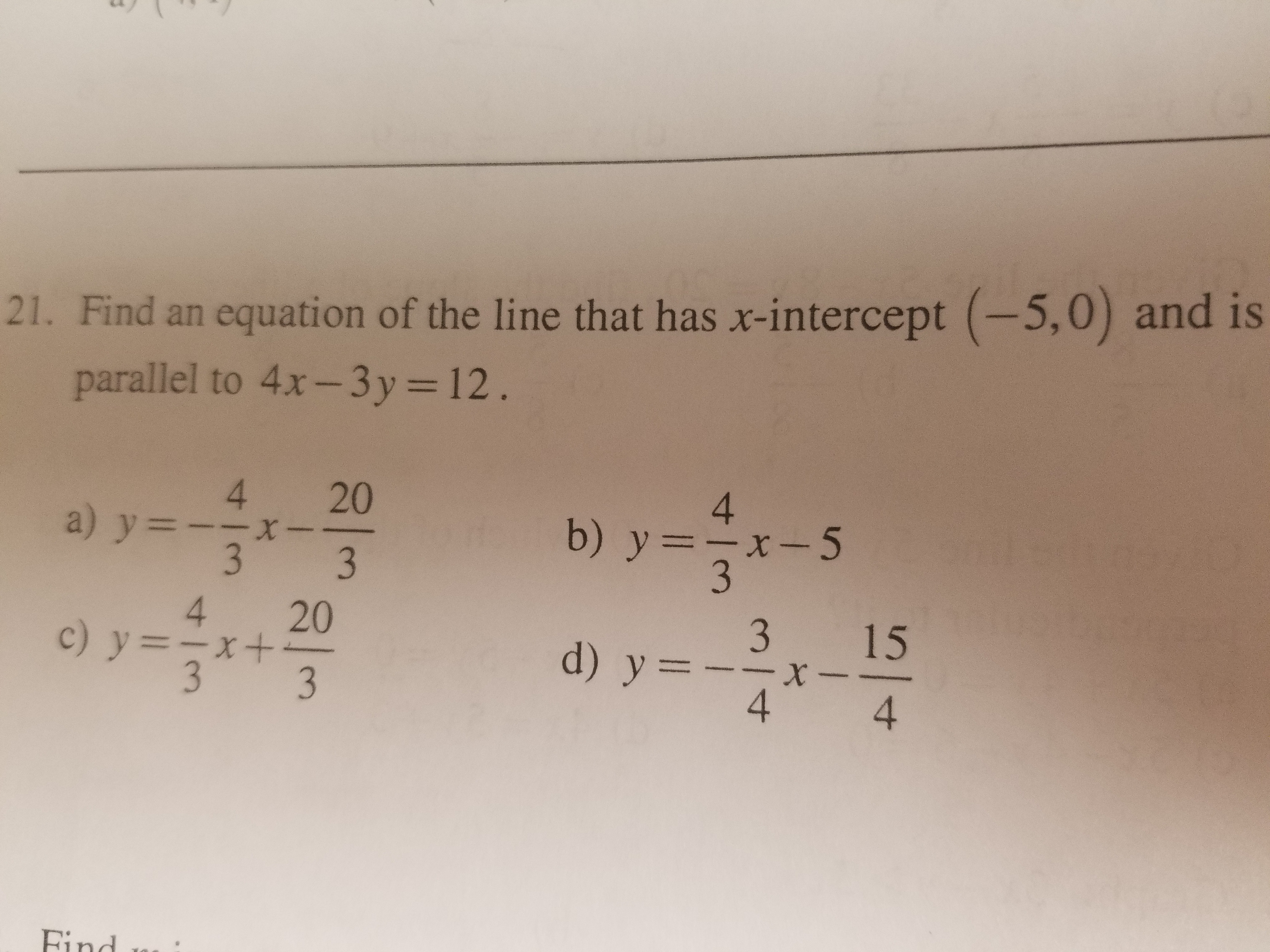21. Find an equation of the line that has x-intercept (-5,0) and is9parallel to 4x-3y 12.4 20b) y-x-54 203 1534d)

Questionhelp_outlineImage Transcriptionclose21. Find an equation of the line that has x-intercept (-5,0) and is 9 parallel to 4x-3y 12. 4 20 b) y-x-5 4 20 3 15 3 4 d) fullscreen
Step 1

Equations of parallel lines are of the form

Given, required equation of line is parallel to 4x-3y = 12

Hence, the required equation of line is of the form 4x-3y = c

Given (-5,0) is the x-intercept of the required line.

Hence, the point satisfies the equation.

Plugging the point in the equation,

Hence, c = -20

Hence, the equation of the line that has x-intercept (-5,0) and is parallel to 4x-3y = 12 is 4x-3y = -20

Step 2

Rewriting the equation of the required line:

Therefore, the equation of the line that has x-intercept (-...

Want to see the full answer?

See Solution

Want to see this answer and more?

Our solutions are written by experts, many with advanced degrees, and available 24/7

See Solution
Tagged in

Equations and In-equations